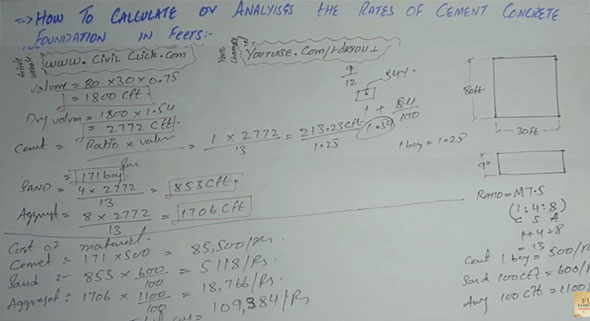#### How to calculate or analyze the rates of cement, fine & course aggregates in a foundation constructionIn this construction video tutorial, one will learn how to work out or analyze the rates of cement, fine aggregates, course aggregates in a foundation construction.

Assume, the length of the foundation is 80 ft and width is 30 ft. The thickness of the foundation is taken as 9 inches. M7.5 concrete grade is used with ratio 1:4:8. (cement : sand : aggregate).

Initially, you have to determine the volume with the following formula :-

Volume = Length x Width x thickness = 80 x 30 x 0.75 (as 9 inches is equal to 0.75 feet) = 1800 cft

It is the wet volume and you have to convert it to dry volume with the following method :-

We know that in measuring unit cubic meter or cubic feet, 54% volume is increased from wet to dry in concrete. To convert from percentage to decimal, just divide by 100 i.e. 1+84/100 = 1.54

So, dry volume = 1800 x 1.54 = 2772 cft

To calculate the quantity of cement, the following formula is used :-

Cement = Ratio x Volume/Sum Of Ratio = 1 x 2772/13 = 213.23 cubic feet

As 1 bag of cement = 1.28 cubic feet

In order to convert it to bags, just divide it with 1.25 i.e. 213.23/1.25 = 171 bags.

Similar formula can be used for calculating the rates of sand and aggregates.

Watch the following video to learn the process for calculating the rates of sand and aggregates.

Video Source: F&U-FORYOU